Module 14 - Related Rates

Introduction | Lesson 1 | Lesson 2 | Lesson 3 | Lesson 4 | Self-Test

This lesson explores related rates by investigating the positions of the foot and the top of a ladder as it slides down a wall.

A 15 foot ladder is held against a wall and then released. The foot of the ladder begins to slide along the ground away from the wall at a constant rate of 3 ft/sec. In the diagram below, the distance from the wall to the foot of the ladder is represented by x, and y represents the distance from the ground to the top of the ladder.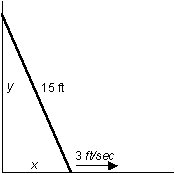• Execute NewProb
• Set the Graph mode to PARAMETRIC

Modeling the Position of the Ladder's Foot

Parametric equations can be used to model the position of the foot of the ladder.

Because the foot of the ladder is moving at a constant rate of 3 ft/sec, it follows that at time t the ladder is 3t from the wall. That is,

x = 3t

Because the foot of the ladder does not rise above the ground and its position along the x-axis is given by 3t, the position of the foot of the ladder can be modeled by the parametric equations given below.

• Set xt1 = 3t
• Set yt1 = 0

Modeling the Position of the Ladder's Top

An expression for the position of the ladder's top, y, can by found by using the Pythagorean theorem.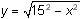The position of the top of the ladder can be modeled by another set of parametric equations.

• Set xt2 = 0
•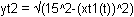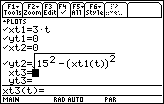Animating the Graphs

• Set the Graph Style of the equations to "Animate" by selecting an equation, opening the Style menu by pressing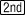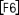and choosing "5:Animate"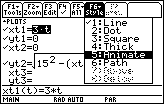• Repeat the process for each pair of equations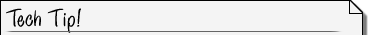Setting Parametric Graph Style The cursor can be on either x or y when changing the graphing style of a pair of parametric equations.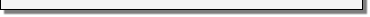Graph the equations simultaneously.

• Select SIMUL for the Graph Order in the Graph Formats dialog box (Pressand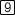)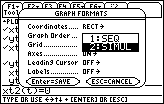• Enter the following window values: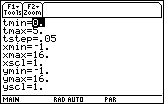• Graph the equations

You should see animated circles that simulate the movement of the top and bottom of the ladder.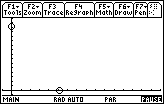You can regraph the equations by pressing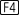.Changing the Animation Speed You can change the apparent speed of the simulation with the value of tstep in the Window editor. Smaller values of tstep slow the simulation, larger values speed it up.Finding the Velocity of the Ladder's Top

How fast is the top moving down the wall when the bottom is 5 ft from the wall?

The instantaneous velocity of the top of the ladder is the value needed, and it is given by the derivative of the function that represents the position of the top. To find the velocity of the top when the bottom is 5 ft from the wall, evaluate the derivative of the position of the top given by yt2 at the value of t that corresponds to x = 5.

Because x = 3t, x = 5 corresponds to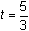. Find the value of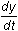when.

• Evaluate d(yt2(t),t) | t=5/3

14.1.2 How fast is the top of the ladder moving when the bottom is 5 ft from the wall? Click here for the answer.

Finding the Velocity Function for the Ladder's Top

The speed of the top of the ladder is a function of time, which is the derivative of its position function.

• Evaluate d(yt2(t),t)